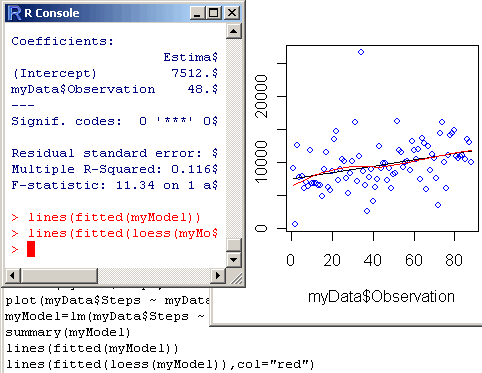Main

## R video tutorial number 2

Filed in Encyclopedia ,RSubscribe to Decision Science News by Email (one email per week, easy unsubscribe)The Decision Science News R video tutorials continue with number 2. (If you missed that last one, you will want to watch R Video Tutorial Number 1 first.) The Goldstein pedometer dataset can be downloaded from http://www.dangoldstein.com/flash/Rtutorial2/pedometer.csv

High Res Version: http://www.dangoldstein.com/flash/Rtutorial2/Rtutorial2.html

Topics covered this week include:

• Tricking R into starting in your working directory
``` myData=read.table("pedometer.csv", header=TRUE, sep=",") x=hist(myData\$Steps,col="lightblue") x=hist(myData\$Steps,breaks=20,col="lightblue") plot(myData\$Steps ~ myData\$Observation,col="blue") myModel=lm(myData\$Steps ~ myData\$Observation) summary(myModel) lines(fitted(myModel)) lines(fitted(loess(myModel)),col="red") ```